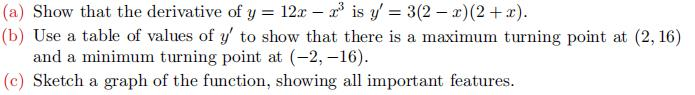### Still have math questions?

Algebra
Question(a) Show that the derivative of $$y = 12 x - x ^ { 3 }$$ is $$y ^ { \prime } = 3 ( 2 - x ) ( 2 + x )$$ .

(b) Use a table of values of $$y ^ { \prime }$$ to show that there is a maximum turning point at $$( 2,16 )$$

and a minimum turning point at $$( - 2 , - 16 )$$ .

(c) Sketch a graph of the function, showing all important features.

-$$2\sqrt{3}$$
$$2\sqrt{3}$$